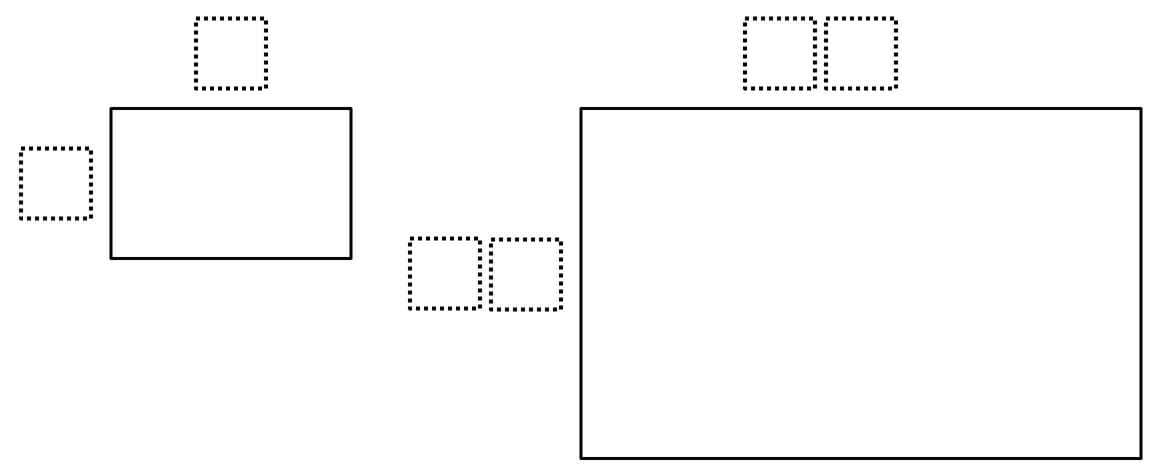Home > Grade 7 > Geometry > Similar Shapes

# Similar Shapes

Directions: Using the digits 0-9, at most one time each, fill in the boxes so that one rectangle is a scaled drawing of the other.### Hint

Enlargements occur when the scale factor is greater than 1.

How many times bigger is the second rectangle compared to the first rectangle?

There are many answers. Here are two of them:

First rectangle is 2 x 8
Second rectangle is 14 x 56
Scale factor would be 7.

First rectangle is 3 x 8
Second rectangle is 15 x 40.
Scale factor would be 5.

Source: Gian Cavaliere

## Converting a Fraction to a Decimal

Directions: Using the digits 1 to 9, at most one time each, place a digit …

1.••